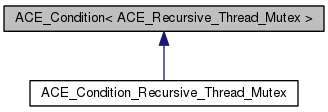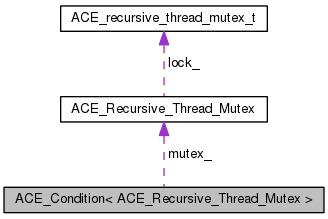ACE  6.0.6

ACE_Condition template specialization written using ACE_Recursive_Thread_Mutex. This allows threads to block until shared data changes state using recursive mutexes. More...

`#include <Condition_Recursive_Thread_Mutex.h>`

Inheritance diagram for ACE_Condition< ACE_Recursive_Thread_Mutex >:[legend]
Collaboration diagram for ACE_Condition< ACE_Recursive_Thread_Mutex >:[legend]

List of all members.

## Public Member Functions

Initialize the condition variable with a recursive mutex.
~ACE_Condition (void)
Implicitly destroy the condition variable.
int remove (void)
int wait (const ACE_Time_Value *abstime=0)
int wait (ACE_Recursive_Thread_Mutex &mutex, const ACE_Time_Value *abstime=0)
int signal (void)
Returns a reference to the underlying mutex;.
void dump (void) const
Dump the state of an object.

## Private Member Functions

void operator= (const ACE_Condition< ACE_Recursive_Thread_Mutex > &)
ACE_Condition (const ACE_Condition< ACE_Recursive_Thread_Mutex > &)

## Private Attributes

ACE_cond_t cond_
A normal (i.e., non-recursive) condition variable.
Reference to the recursive mutex.

## Detailed Description

ACE_Condition template specialization written using ACE_Recursive_Thread_Mutex. This allows threads to block until shared data changes state using recursive mutexes.

## Constructor & Destructor Documentation

Initialize the condition variable with a recursive mutex.

 ACE_Condition< ACE_Recursive_Thread_Mutex >::~ACE_Condition ( void )

Implicitly destroy the condition variable.

 ACE_Condition< ACE_Recursive_Thread_Mutex >::ACE_Condition ( const ACE_Condition< ACE_Recursive_Thread_Mutex > & ) ` [private]`

## Member Function Documentation

 void ACE_Condition< ACE_Recursive_Thread_Mutex >::dump ( void ) const

Dump the state of an object.

Returns a reference to the underlying mutex;.

 void ACE_Condition< ACE_Recursive_Thread_Mutex >::operator= ( const ACE_Condition< ACE_Recursive_Thread_Mutex > & ) ` [private]`
 int ACE_Condition< ACE_Recursive_Thread_Mutex >::remove ( void )

Explicitly destroy the condition variable. Note that only one thread should call this method since it doesn't protect against race conditions.

 int ACE_Condition< ACE_Recursive_Thread_Mutex >::signal ( void )

 int ACE_Condition< ACE_Recursive_Thread_Mutex >::wait ( const ACE_Time_Value * abstime = `0` )

Block on condition, or until absolute time-of-day has passed. If abstime == 0 use "blocking" <wait> semantics. Else, if abstime != 0 and the call times out before the condition is signaled <wait> returns -1 and sets errno to ETIME.

 int ACE_Condition< ACE_Recursive_Thread_Mutex >::wait ( ACE_Recursive_Thread_Mutex & mutex, const ACE_Time_Value * abstime = `0` )

Block on condition or until absolute time-of-day has passed. If abstime == 0 use "blocking" wait() semantics on the recursive mutex passed as a parameter (this is useful if you need to store the <Condition> in shared memory). Else, if <abstime> != 0 and the call times out before the condition is signaled <wait> returns -1 and sets errno to ETIME.

## Member Data Documentation

 ACE_cond_t ACE_Condition< ACE_Recursive_Thread_Mutex >::cond_` [private]`

A normal (i.e., non-recursive) condition variable.

 ACE_Recursive_Thread_Mutex& ACE_Condition< ACE_Recursive_Thread_Mutex >::mutex_` [private]`

Reference to the recursive mutex.

The documentation for this class was generated from the following files: